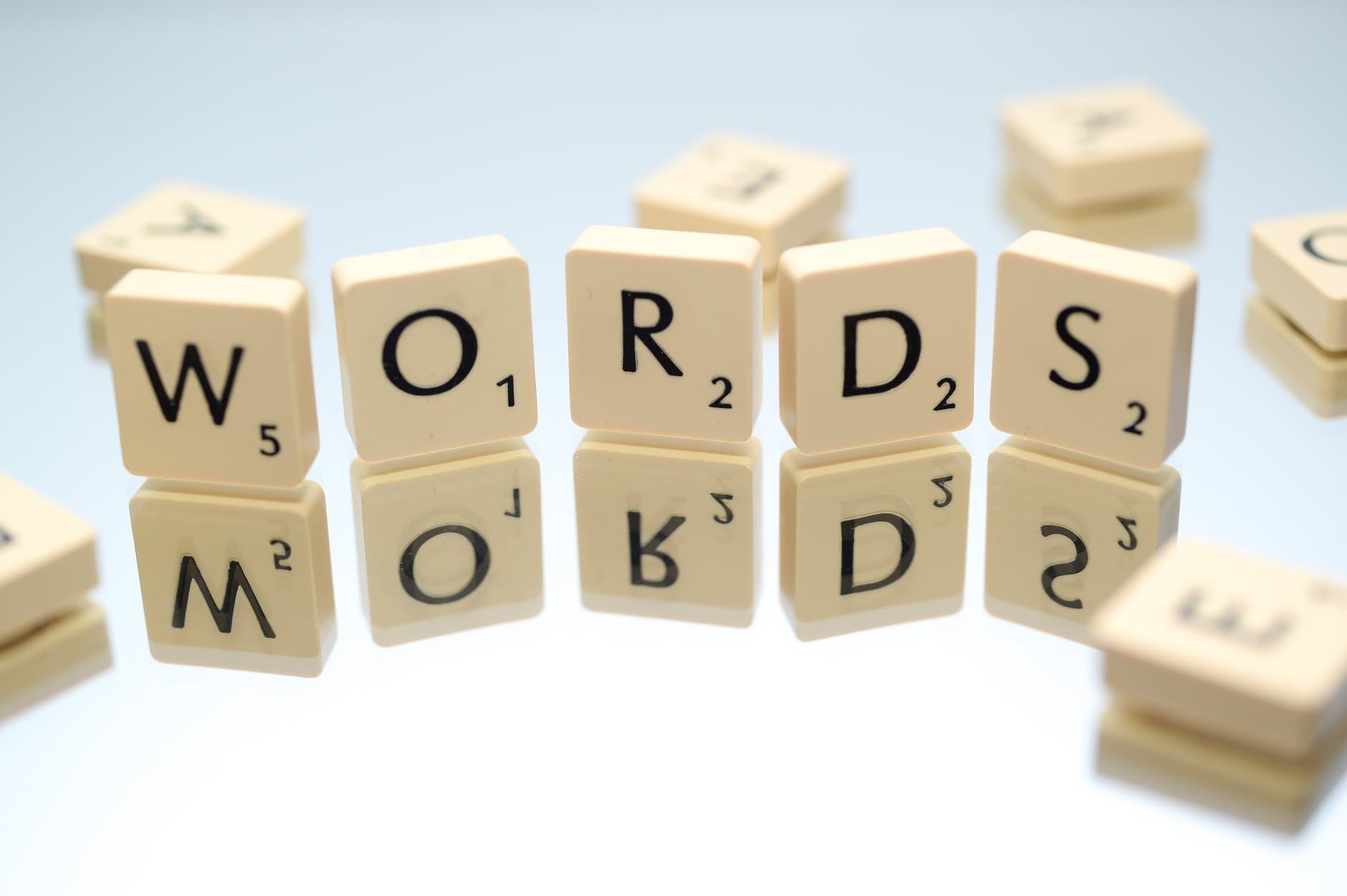# String Functions and Excel VBA

This post will provide several examples of using string functions in the Excel VBA developer. There are a large number of string functions that are available in Excel but we are only going to look at the following…

• InStr
• UCase
• LCase
• Mid
• Join

The first example will use most of the functions listed above. Below is the code for the subroutine we are creating.

``````Sub modString()
Dim text As String, text2 As String
text = "educationalresearchtechniques"
text2 = "EDUCATIONALRESEARCHTECHNIQUES"
MsgBox InStr(text, "cat")
MsgBox InStr(10, text, "i")
MsgBox UCase(text)
MsgBox LCase(text2)
End Sub``````

This code is rather straightforward. We are manipulating the phrase “educationalresearchtechniques” or the same phrase in all caps.

The InStr function takes the text variable and searches it for the string “cat.” The output gives the position at which you can find the phrase “cat”. Below is the output from the message box.

The string “cat” begins in the fourth position of “educationalresearchtechniques” which you can see for yourself.

The second InStr function tells VBA to skip the first 10 spaces in the string and then look for the letter “i”. This was done because the letter i appears twice in the string so this allows you to find the second appearance of the letter “i”. Below is the output.

The second “i” appears in the 25th position of the string.

The last two functions are similar. The UCase function makes all letters capitals and the LCase function makes all letters lower case. Below we have both outputs shown.

The output to the left uses the variable “text” while the output to the right used the variable “text2.”

Arrays

The coding for the array is more complex. Our goal is to remove the spaces when we join the text in an array. Below is the first part of the code

``````Option Base 1
Sub joinText()
Dim word(4) As String, combineMsg As String, msg As String
word(1) = "z"
word(2) = "ys"
word(3) = "x"
word(4) = "w"
combineMsg = Join(word)``````

In the code below we declare several variables that we will need and we add the content to the array we created called “word.” This array is a 1 x 4 array. Each time we type word(x) we are putting the string after the equal sign in that position. A the bottom of the code we use a Join function to combine all the strings in the array into one string. The problem is that this string has spaces between the letters. To fix this, we will use the for loop and if-then statement below.

``````For i = 1 To Len(combineMsg)
If Not Mid(combineMsg, i, 1) = " " Then
msg = msg + Mid(combineMsg, i, 1)
End If
Next i
MsgBox (msg)
End Sub``````

This for loop goes from i to the length of the combineMsg string. In the next line, we use the mid function to tell VBA to start looking at whatever ith position we are at and to take one character from the string. If the character is not a space ” ” then we will add it to the new variable called msg. If it is a space we ignore it. Finally, after this has looped several times we exit and print the new string in a message box. The output is below.

What you see above is the locals window from the VBA developer. You can see that the combineMsg variable has spaces between the input from the word array. However, this is fixed when you look at the msg variable in the next line. Below is the output from the Message Box.

Conclusion

String functions are another tool that has many applications in Excel VBA. We only touch on some basic ideas here but there is so much more that can be done.